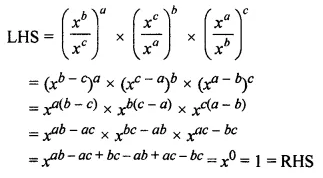# ML Aggarwal Class 7 Solutions for ICSE Maths Chapter 4 Exponents and Powers Objective Type Questions

## ML Aggarwal Class 7 Solutions for ICSE Maths Chapter 4 Exponents and Powers Objective Type Questions

Mental Maths

Question 1.
Fill in the blanks:
(i) In the expression (-5)9, exponent = ……… and base = ………..
(ii) If the base is $$\frac { -3 }{ 4 }$$ and exponent is 5, then exponential form is ………
(iii) The expression (x2y5)3 in the simplest form is ………
(iv) If (100)0 = 10n, then the value of n is ……..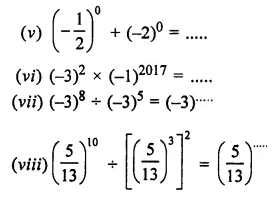(ix) 35070000 = 3.507 × 10….
(x) If (-2)n = -128, then n = ……..
Solution: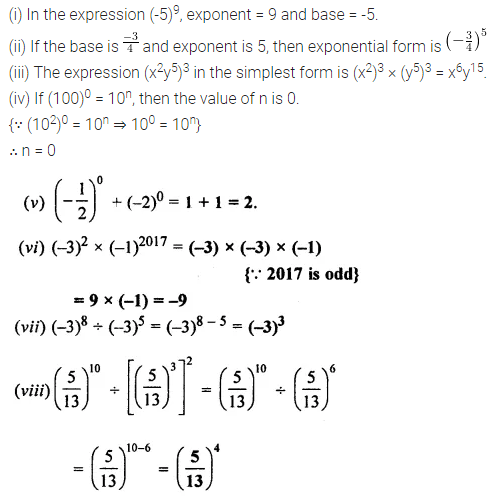Question 2.
State whether the folloiwng statements are true (T) or false (F):
(i) If a is a rational number then am × an = am×n
(ii) 23 × 32 = 65.
(iii) The value of (-2)-3 is $$\frac { -1 }{ 8 }$$.
(iv) The value of the expression 29 × 291 – 219 × 281 is 1.
(v) 30 = (1000)0.
(vi) 56 ÷ (-2)6 = $$\frac { -5 }{ 2 }$$
(vii) 50 × 30 = 80
(viii) $$\frac { { 2 }^{ 3 } }{ 7 } <\left( \frac { 2 }{ 7 } \right) ^{ 3 }$$
(ix) (10 + 10)4 = 104 + 104.
(x) x0 × x0 = x0 ÷ x0, where x is a non-zero rational number.
(xi) 49 is greater than 163.
(xii) xm + xm = x2m, where x is a non-zero rational number and m is a positive integer.
(xiii) $$\left( \frac { 4 }{ 3 } \right) ^{ 5 }\times \left( \frac { 5 }{ 7 } \right) ^{ 5 }=\left( \frac { 4 }{ 3 } +\frac { 5 }{ 7 } \right) ^{ 5 }$$
Solution: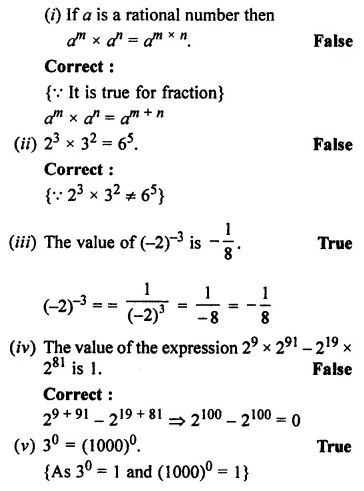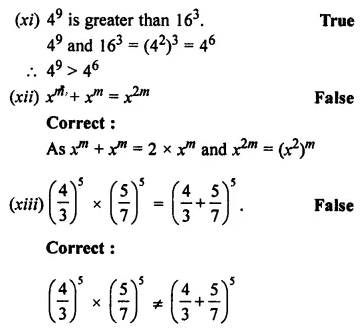Multiple Choice Questions

Choose the correct answer from the given four options (3 to 18):
Question 3.
a × a × a × b × b × b is equal to
(a) a3b2
(b) a2b3
(c) (ab)3
(d) a6b6
Solution:Question 4.
(-2)3 × (-3)2 is equal to
(a) 65
(b) (-6)6
(c) 72
(d) -72
Solution: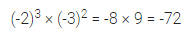Question 5.
The expression (pqr)3 is equal to
(a) p3qr
(b) pq3r
(c) pqr3
(d) p3q3r3
Solution: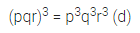Question 6.Solution: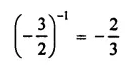Question 7.Solution:Question 8.
The value of (530 × 520) ÷ (55)9 in the exponential form is
(a) 5-5
(b) 55
(c) 550
(d) 595
Solution:Question 9.
The law $$\left( \frac { a }{ b } \right) ^{ n }=\frac { { a }^{ n } }{ b^{ n } }$$ does not hold when
(a) a = 3, b = 2
(b) a = -2, b = 3
(c) n = 0
(d) b = 0
Solution: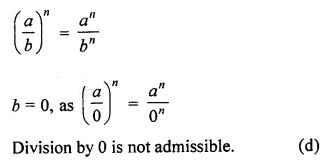Question 10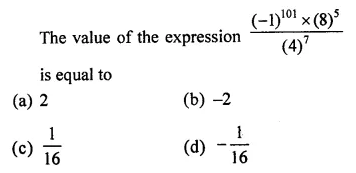Solution: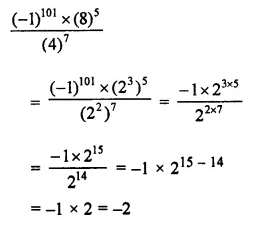Question 11.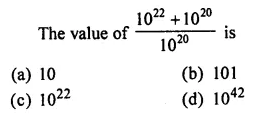Solution: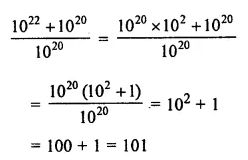Question 12.
The value of 5-1 – 6-1 is
(a) $$\frac { 1 }{ 30 }$$
(b) $$\frac { -1 }{ 30 }$$
(c) 30
(d) -30
Solution:Question 13.
The value of (6-1 – 8-1)-1 is
(a) $$\frac { -1 }{ 2 }$$
(b) -2
(c) $$\frac { 1 }{ 24 }$$
(d) 24
Solution: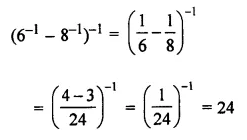Question 14.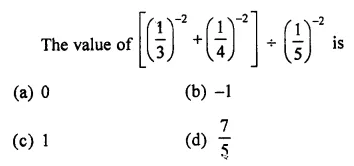Solution: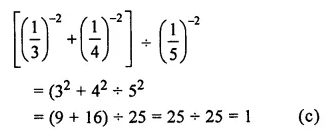Question 15.
If 23 + 13 = 3x, then the value of x is
(a) 0
(b) 1
(c) 2
(d) 3
Solution: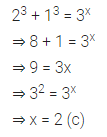Question 16.
The standard form of 751.65 is
(a) 7.5165 × 102
(b) 75.165 × 101
(c) 7.5165 × 104
(d) 7.51 × 102
Solution:Question 17.
The usual form of 5.658 × 105 is
(a) 5658
(b) 56580
(c) 565800
(d) 5658000
Solution: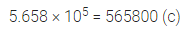Question 18.
Which of the following numbers is in the standard form?
(a) 26.57 × 104
(b) 2.657 × 104
(c) 265.7 × 103
(d) 0.2657 × 106
Solution: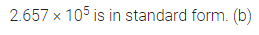Value Based Questions

Question 1.
Typhoid is caused by bacteria Salmonella typhi. The size of Salmonella typhi is about 0.0000000005 mm. Express it in standard form. Vinay is suffering from typhoid, his doctor advised him to take healthy food and avoid eating food or drinking beverages from street vendors.
Why should we eat healthy food and why should we not eat food from street vendors?
Solution:Higher Order Thinking Skills (HOTS)

Question 1.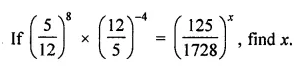Solution: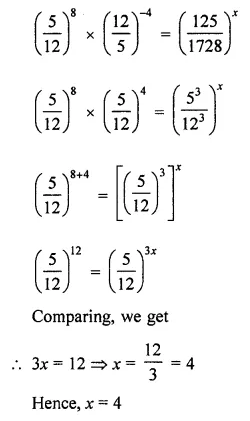Question 2.Solution: